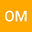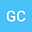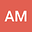Neutral delay differential equations: An improved approach and its applications in the oscillation theory
•••• Osama Moaaz,
• George Chatzarakis,
• Ali Muhib
Osama Moaaz
Mansoura University Faculty of Science

Corresponding Author:[email protected]

Author ProfileGeorge Chatzarakis
School of Pedagogical and Technological Education
Author ProfileAli Muhib
Ibb University
Author Profile## Abstract

The objective of this study is to establish new sufficient criteria for the oscillation of the 2nd-order neutral equation $\left( r\left( z^{\prime }\right) ^{\alpha }\right) ^{\prime }\left( t\right) +q\left( t\right) x^{\beta }\left( \sigma \left( t\right) \right) =0,$ where $t\geq t_{0}$ and $z\left( t\right) =x\left( t\right) +px\left( \tau \left( t\right) \right)$%. We improve the known criteria by establishing a new relationship between the solution $x$ and the corresponding function $z$. To show the importance of our results, we provide two examples.